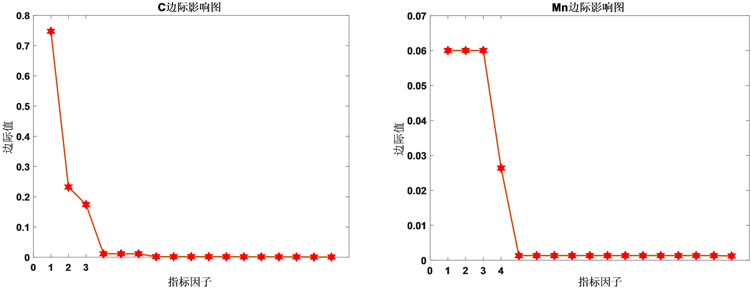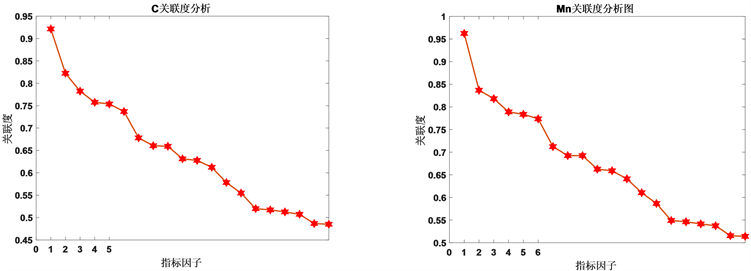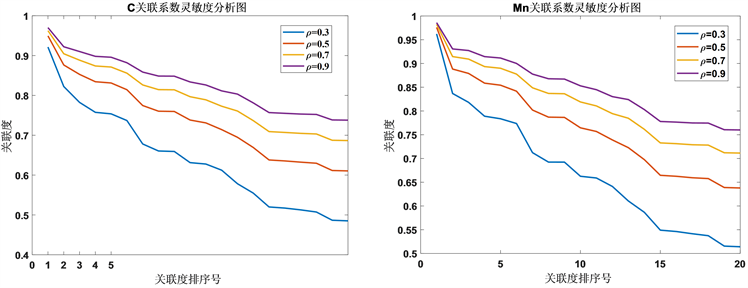1 ( W s + W i ) C 0 W s W i δ iTable 1. Single factor analysis of variance

3. 边际影响模型

$\frac{\partial Y}{\partial {W}_{s}}=\frac{\left({C}_{1}-{C}_{0}\right)}{\sum {W}_{i}{\delta }_{i}}$

$\frac{\partial Y}{\partial {W}_{i}}=\frac{{C}_{1}\sum {W}_{i}{\delta }_{i}-\left(\left({C}_{1}-{C}_{0}\right){W}_{s}+{C}_{1}\sum {W}_{i}\right){\delta }_{i}}{{\sum {W}_{i}{\delta }_{i}}^{\text{​}}}$Figure 1. Marginal influence diagram of indicators on carbon (left) and manganese (right) element yieldsTable 2. Ranking table of marginal impact of factors affecting carbon and manganese

4. 灰色关联度模型

${Q}_{o}=\left\{{Q}_{o}\left(k\right)|k=1,2,\cdots ,n\right\}=\left({Q}_{o}\left(1\right),{Q}_{o}\left(2\right),\cdots ,{Q}_{o}\left(n\right)\right)$

${Q}_{j}=\left\{{Q}_{j}\left(k\right)|k=1,2,\cdots ,n\right\}=\left({Q}_{j}\left(1\right),{Q}_{j}\left(2\right),\cdots ,{Q}_{j}\left(n\right)\right),j=1,2,\cdots ,23$

${r}_{i}\left(k\right)=\frac{\underset{s}{\mathrm{min}}\underset{t}{\mathrm{min}}|{Q}_{0}\left(t\right)-{Q}_{s}\left(t\right)|+\rho \underset{s}{\mathrm{max}}\underset{t}{\mathrm{max}}|{Q}_{0}\left(t\right)-{Q}_{s}\left(t\right)|}{|{Q}_{0}\left(t\right)-{Q}_{i}\left(t\right)|+\rho \underset{s}{\mathrm{max}}\underset{t}{\mathrm{max}}|{Q}_{0}\left(t\right)-{Q}_{s}\left(t\right)|}$

$Co{r}_{i}=\frac{1}{n}\underset{k=1}{\overset{n}{\sum }}{r}_{i}\left(k\right)$Figure 2. Relevance analysis of carbon (left) and manganese (right) elementsTable 3. Ranking of correlation degree of influencing factors of carbon and manganese recovery

5. 灰色关联度模型的灵敏度检验

6. 结语Figure 3. Sensitivity analysis diagram based on correlation coefficient

  陈襄武. 炼钢过程的脱氧[M]. 北京: 冶金工业出版社, 1991.  李丰功, 战东平, 张佩灿, 等. 脱氧合金化工艺对高铝钢中夹杂物的影响[J]. 中国冶金, 2013, 23(7): 21-23.  林鹏程, 吴启勋. 灰色关联度分析及应用[J]. 盐湖研究, 2001, 9(2): 48-50.  杨怀春, 马进国, 姜国才. 碳化硅对转炉炼钢脱氧合金化的影响分析[J]. 新疆钢铁, 2014(2): 43-45.